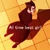## FANDOM

25,441 Pages# RatherClueless

## aka Rather, recently also Clueless

0 Discussion posts
• I live in Beeing nosy, are we?
• My occupation is Why are you asking?
• I am What difference does it make?
•## Explosion Physics

November 27, 2019 by RatherClueless

The amount of joules you tank is:

(J_1/s*A_1*r_4*v(total))/(4*pi*(r_2)^2)=J_2

J_1 is the initial ammount of joules (black box eplosion values)

J_2 are the actually tanked joules

A_1 Area of object (A2s surface area)

r_2 is the distance from center of explosion to object

r_4=(r_2-cbrt((r_2)^3-(r_1)^3))

r_1 is the original radius of the energy density holding object

v(total) is the velocity of expansion over the distance of r_2 to r_2+r_4

v(total)=(1/r_4)*[(x'*g*(r_2+r_4))/(e^(r_2+r_4*g/(v_inf)^2)*(v_inf)^2-(v_inf)^2)] (r_2 to r_2+r_4)

x' is the intitial velocity of expansion

g is acceleration due to gravity

(v_inf)^2 is m*g/k

m=4/3*pi*A'^(3/2)*p_1 and is the mass A'=(1/r_4)*[4*pi*x^2](r_2 to r_2+r_4) +4*pi*(r_2-r_1)^2 and is the Area

p_1 is the density …

•## Stelly blowing away the clouds

August 29, 2019 by RatherClueless

Stella blows away a bunch of clouds with her flames in a fight.

It is stated that she could have spread her flames all over Osaka.

Her flames are said to be as hot as the core of the sun (15,000,000°C).

For the low end I'll go with 3000°C

So to get started I'll assume 2km height for the clouds, since that will be the height her flames can reach to. The area she could affect is all of Osaka. Osakas area, depending on where you look, ranges from 221km^2 to 225km^2, so I'll go with 223km^2.

The specific heat capacity of air is 1.005kj/Kg.K.

Average Japanese summer temerature is roughly 25°C

Density of orange fire: 0.3kg/m^3

Volume: 221km^2*2km=4.46*10^11m^3

Mass: 4.46*10^11m^3*0.3kg/m^3=1.338*10^11kg

Change of temperature (1): 2975°C

Change of temperatu…

•## Everything about Iruna and Toram

August 22, 2019 by RatherClueless

Toram and Iruna are two mobile MMORPGS made by Asmomibo. They take place in the universe Iruna and focus around an adventurer who wanders through the world looking for exciting things to do.

In this blog I don't want to cover pretty much anythingthat is related to the game, may it be the lore, game mechanics or items and equipments.

Iruna is a world created by the 12 gods. One of the 12 gods, Specia, the godess of species, was said to have been so beutiful that all male gods waged war over her. Specia, who couldn't stand to see the suffering decided to take her own life. After that, the war came to a quick stop. Obligaus, the god of justice resigned from his position as god and Grecia, the godess of courage was exiled from the gods due to no…

•## Kanbaru smashes ground

August 12, 2019 by RatherClueless

I used two different ways to get the size of the crater:

Kanbaru is 160cm

red line (118.6086px) + blue line (127.0472353) + purple line (58.52349955) = 304.1793349px

1.6m = 304.1793349px

1px = 0.005260054897m

1px^3 = 1.45536133×10^−7m^3

Radius (sphere): 337.6462646px (equal to 1071.333096px in picture 1)

Radius (cap): 926.5px (picture 1)/ 146px (picture 2)

px factor is 6.34589041,

Crater depth via px-scaling: 193px

Angle: 52° (measuring)

Angle: 47.06° (calculation after px-scaling)

Useing the spherical cap sector calculator we get 305859274.2px^3 (angle)/264000575.98px^3 (px-scaling) for the volume of the cap.

Via angle:

305859274.2px^3 = 44.51357601m^3

Via px-scaling:

264000575.98px^3 = 38.42162294m^3

Note: I also calced the size as an ellipsoid and got a…

•## Vaporizing Sofya city

July 20, 2019 by RatherClueless

So basically there is a guy who uses a weapon of mass destruction, that could vaporize a city called "Sofya city" within an instant, so I wnt ahead and tried my best to measure and calc it.

I know it says "blow up", but the guy who build it specificly states that it uses vaporization.

• red: 508.284359px
• green: 367.164812px

I started by measuring the size of the adventurer in Toram, since that is the only game where we get an actual distance.

I then scaled the sizes over to Iruna using one of the bosses.

• red: 125.606528px
• green: 135.003703px
• blue: 117.643529px
• yellow: 359.012534px

r+g+b: 378.2537615px

To then end up with a size of 1.384845403m

Note: Trying to get the size was rly hard, since the mobs keep running around and because 2m-2.99m is a…